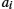Appendix F

# Finite Element Matrices

## 6.1 Shape Functions Over Triangle

Shape functions expanded over a triangular element can be expressed using a polynomial

${N}_{i}\left(x,y,z\right)={a}_{i}+{b}_{i}x+{c}_{i}y,i=1,2,3,$(F.1)

where ${a}_{i}$, ${b}_{i}$and ${c}_{i}$are the coefficients to be found [1,2]. Since the shape function is of local support, it is convenient to derive it using the so-called simplex ...

Get Human Interaction with Electromagnetic Fields now with the O’Reilly learning platform.

O’Reilly members experience live online training, plus books, videos, and digital content from nearly 200 publishers.